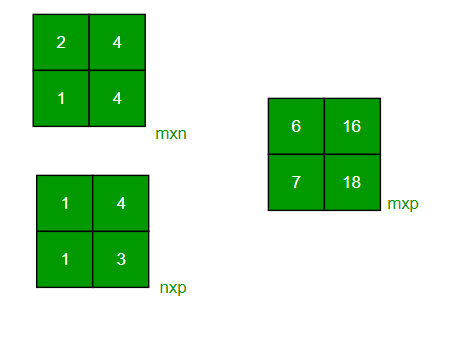# Java Program to Multiply two Matrices of any size

• Difficulty Level : Easy
• Last Updated : 23 Apr, 2022

Given two matrices A and B of any size, the task to multiply them in Java.
Examples:

```Input: A[][] = {{1, 2},
{3, 4}}
B[][] = {{1, 1},
{1, 1}}
Output: {{3, 3},
{7, 7}}

Input: A[][] = {{2, 4},
{3, 4}}
B[][] = {{1, 2},
{1, 3}}
Output: {{6, 16},
{7, 18}}```

Approach:

• Take the two matrices to be multiplied
• Check if the two matrices are compatible to be multiplied• Create a new Matrix to store the product of the two matrices
• Traverse each element of the two matrices and multiply them. Store this product in the new matrix at the corresponding index.
• Print the final product matrix

Below is the implementation of the above approach:

## Java

 `// Java program to multiply two square matrices.` `import` `java.io.*;` `class` `GFG {` `    ``// Function to print Matrix``    ``static` `void` `printMatrix(``int` `M[][],``                            ``int` `rowSize,``                            ``int` `colSize)``    ``{``        ``for` `(``int` `i = ``0``; i < rowSize; i++) {``            ``for` `(``int` `j = ``0``; j < colSize; j++)``                ``System.out.print(M[i][j] + ``" "``);` `            ``System.out.println();``        ``}``    ``}` `    ``// Function to multiply``    ``// two matrices A[][] and B[][]``    ``static` `void` `multiplyMatrix(``        ``int` `row1, ``int` `col1, ``int` `A[][],``        ``int` `row2, ``int` `col2, ``int` `B[][])``    ``{``        ``int` `i, j, k;` `        ``// Print the matrices A and B``        ``System.out.println(``"\nMatrix A:"``);``        ``printMatrix(A, row1, col1);``        ``System.out.println(``"\nMatrix B:"``);``        ``printMatrix(B, row2, col2);` `        ``// Check if multiplication is Possible``        ``if` `(row2 != col1) {` `            ``System.out.println(``                ``"\nMultiplication Not Possible"``);``            ``return``;``        ``}` `        ``// Matrix to store the result``        ``// The product matrix will``        ``// be of size row1 x col2``        ``int` `C[][] = ``new` `int``[row1][col2];` `        ``// Multiply the two matrices``        ``for` `(i = ``0``; i < row1; i++) {``            ``for` `(j = ``0``; j < col2; j++) {``                ``for` `(k = ``0``; k < row2; k++)``                    ``C[i][j] += A[i][k] * B[k][j];``            ``}``        ``}` `        ``// Print the result``        ``System.out.println(``"\nResultant Matrix:"``);``        ``printMatrix(C, row1, col2);``    ``}` `    ``// Driver code``    ``public` `static` `void` `main(String[] args)``    ``{` `        ``int` `row1 = ``4``, col1 = ``3``, row2 = ``3``, col2 = ``4``;` `        ``int` `A[][] = { { ``1``, ``1``, ``1` `},``                      ``{ ``2``, ``2``, ``2` `},``                      ``{ ``3``, ``3``, ``3` `},``                      ``{ ``4``, ``4``, ``4` `} };` `        ``int` `B[][] = { { ``1``, ``1``, ``1``, ``1` `},``                      ``{ ``2``, ``2``, ``2``, ``2` `},``                      ``{ ``3``, ``3``, ``3``, ``3` `} };` `        ``multiplyMatrix(row1, col1, A,``                       ``row2, col2, B);``    ``}``}`

Output:

```Matrix A:
1 1 1
2 2 2
3 3 3
4 4 4

Matrix B:
1 1 1 1
2 2 2 2
3 3 3 3

Resultant Matrix:
6 6 6 6
12 12 12 12
18 18 18 18
24 24 24 24```

Time Complexity: O(M2*N), as we are using a nested loop for traversing.

Auxiliary Space: O(M*N), as we are using extra space.

My Personal Notes arrow_drop_up